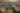# Infinite Horizon Gaussian Processes

Date August 25, 2020
Authors Arno Solin (Aalto Yliopisto), Richard Turner (University of Cambridge), James Hensman (Secondmind)

Gaussian processes provide a flexible framework for forecasting, removing noise, and interpreting long temporal datasets. State space modelling (Kalman filtering) enables these non-parametric models to be deployed on long datasets by reducing the complexity to linear in the number of data points. The complexity is still cubic in the state dimension m. In certain special cases (Gaussian likelihood, regular spacing) the GP posterior will reach a steady posterior state when the data is very long. We leverage this and formulate an inference scheme for GPs with general likelihoods, where inference is based on single-sweep EP (assumed density filtering). The infinite-horizon model tackles the cubic cost in the state dimensionality and reduces the cost in the state dimension m to O(m2) per data point. The model is extended to online-learning of hyperparameters. We show examples for large finite-length modelling problems, and present how the method runs in real-time on a smartphone on a continuous data stream updated at 100 Hz Infinite Horizon Gaussian Processes.

View the paper

Share
,,